# BFS Given an undirected graph below (a) Show the shortest distance to each vertex from source ver...

Solve (a) and (b) using BFS and DFS diagram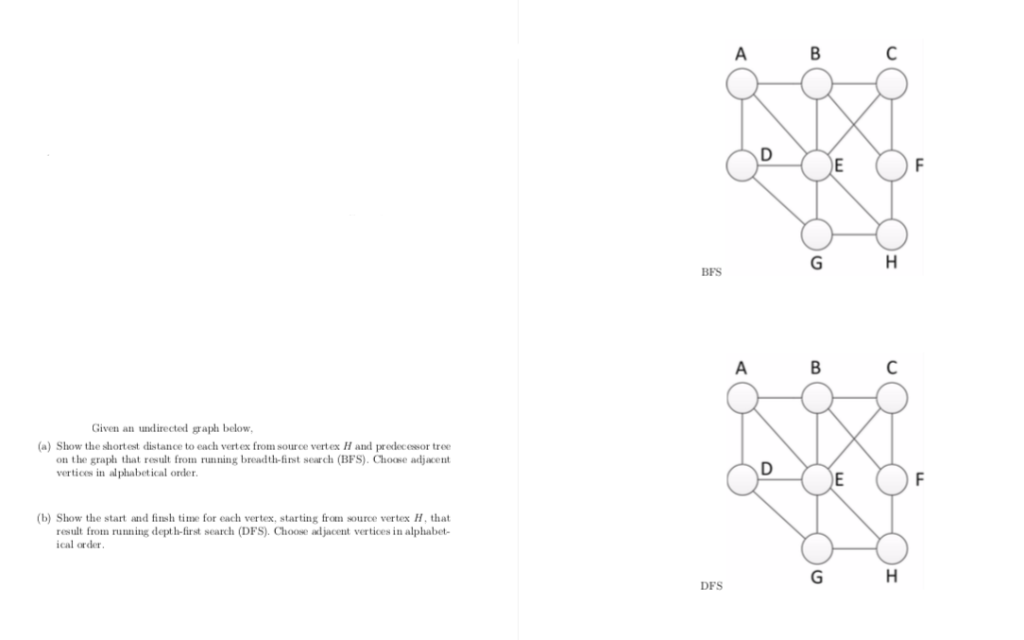BFS Given an undirected graph below (a) Show the shortest distance to each vertex from source vertex H and predecessor tree on the graph that result from running breadth-finst search (BFS).Choose adjacen vertices in al phabetical order b) Show the start and finsh time for each vertex, starting from source vertex H, that result from running depth-first search (DFS)Choose aljacent vertices in alphabet- ical order DFS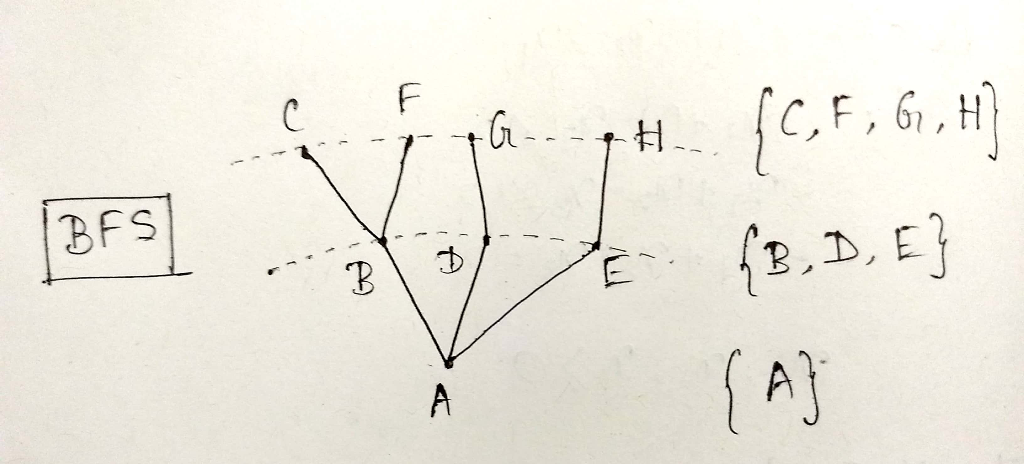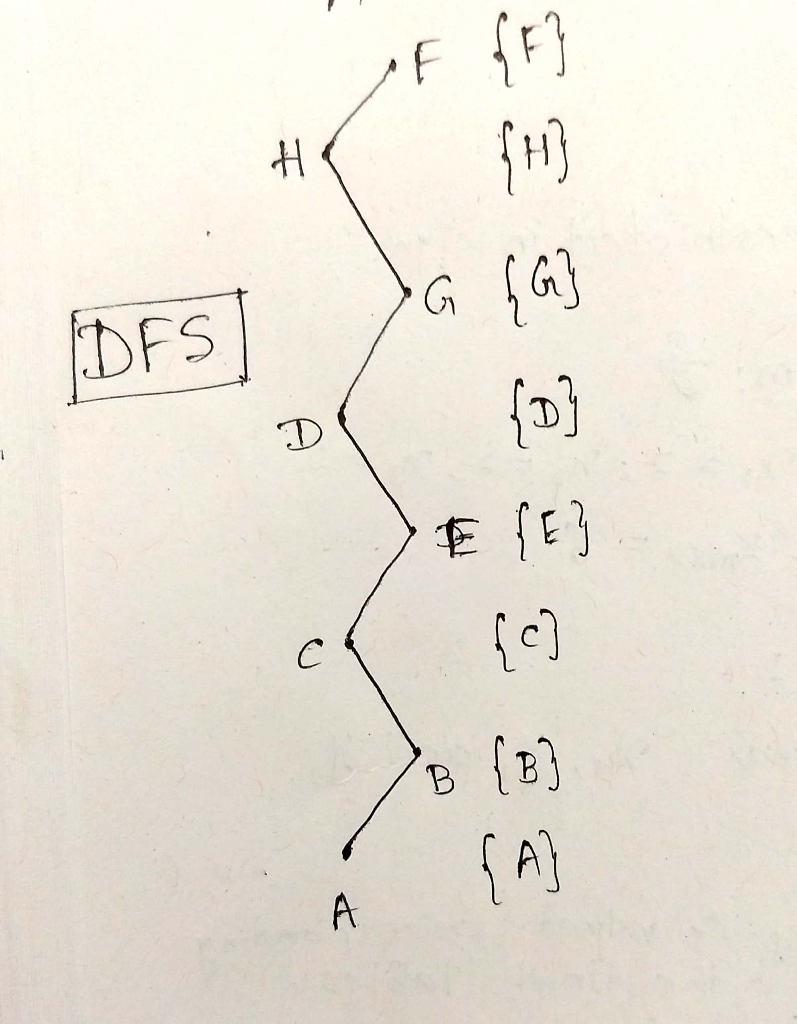#### Earn Coin

Coins can be redeemed for fabulous gifts.

Similar Homework Help Questions
• ### BFS Given an undirected graph below (a) Show the shortest distance to each vertex from source ver...BFS Given an undirected graph below (a) Show the shortest distance to each vertex from source vertex H and predecessor tree on the graph that result from running breadth-finst search (BFS).Choose adjacen vertices in al phabetical order b) Show the start and finsh time for each vertex, starting from source vertex H, that result from running depth-first search (DFS)Choose aljacent vertices in alphabet- ical order DFS BFS Given an undirected graph below (a) Show the shortest distance to each vertex...

• ### Show the operation of depth-first search (DFS) on the graph of Figure 1 starting from vertex...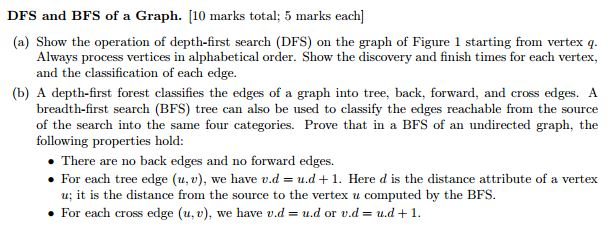Show the operation of depth-first search (DFS) on the graph of Figure 1 starting from vertex q. Always process vertices in alphabetical order. Show the discovery and finish times for each vertex, and the classification of each edge. (b) A depth-first forest classifies the edges of a graph into tree, back, forward, and cross edges. A breadth-first search (BFS) tree can also be used to classify the edges reachable from the source of the search into the same four categories....

• ### For the following graph: BFS (a) Perform BFS on the following graph starting at vertex m...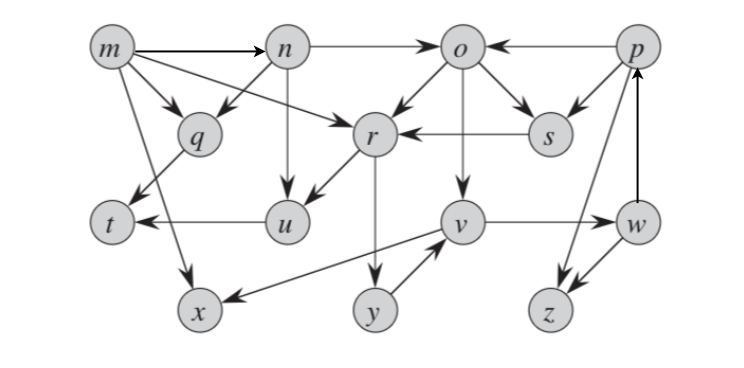For the following graph: BFS (a) Perform BFS on the following graph starting at vertex m show v.d and v.π for each vertex. (b) Draw the Breadth first predecessor tree resulting from running the algorithm in part (a).

• ### From the given graph discover the structure of the graph using 1. breadth first search(BFS) a. depth first search(DFS) b. Show the steps and techniques used for each method (20 points) From the...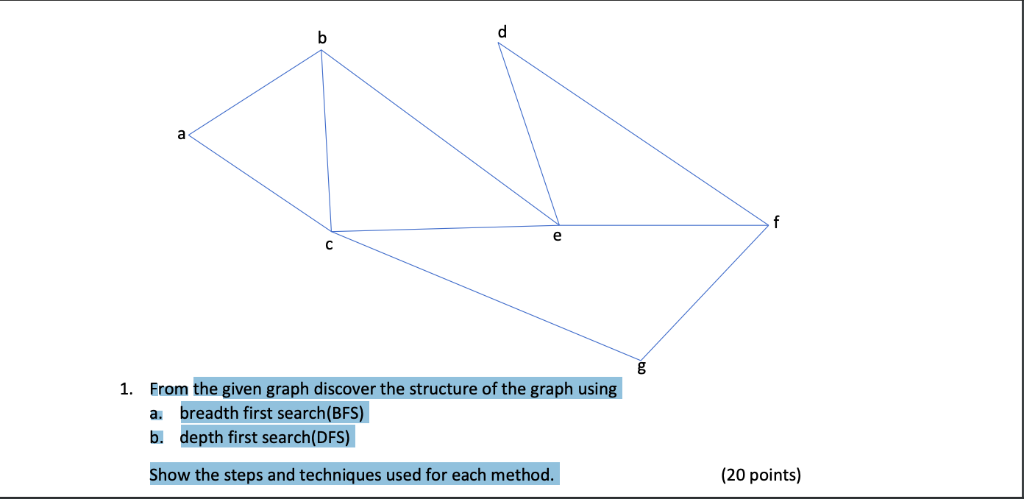From the given graph discover the structure of the graph using 1. breadth first search(BFS) a. depth first search(DFS) b. Show the steps and techniques used for each method (20 points) From the given graph discover the structure of the graph using 1. breadth first search(BFS) a. depth first search(DFS) b. Show the steps and techniques used for each method (20 points)

• ### 3. (8 points-7+1) Figure 4 shows an undirected graph G. Assume that the adjacency list lists the ...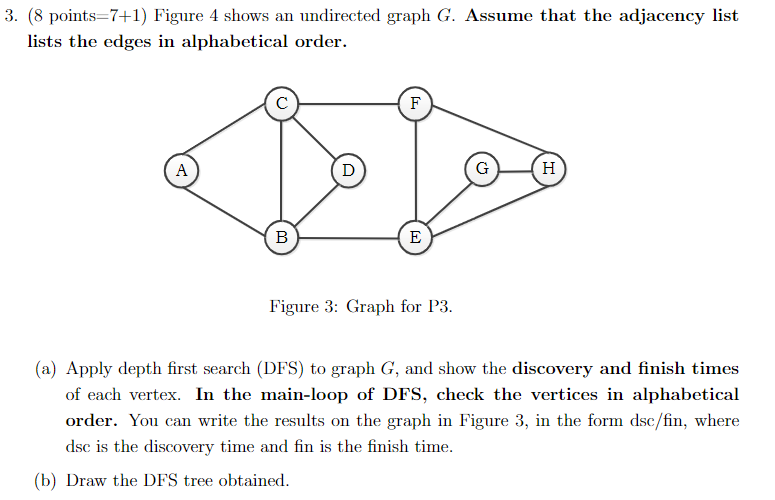3. (8 points-7+1) Figure 4 shows an undirected graph G. Assume that the adjacency list lists the edges in alphabetical order. Figure 3: Graph for P3 (a) Apply depth first search (DFS) to graph G, and show the discovery and finish times of each vertex. In the main-loop of DFS, check the vertices in alphabetical the form dsc/fin, where dsc is the discovery time and fin is the finish time. (b) Draw the DFS tree obtained. 3. (8 points-7+1) Figure...

• ### please I need it urgent thanks algorithms second picture is the graph 2.3 Graphs and BFS-DFS...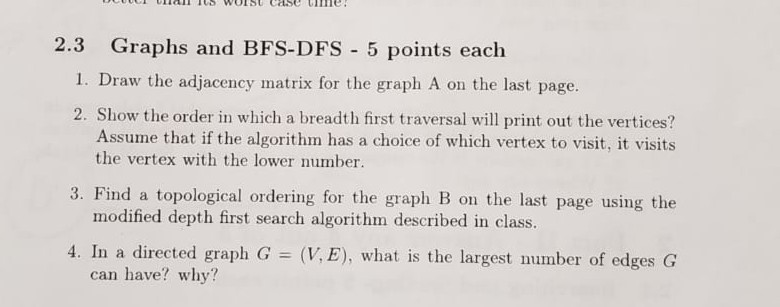please I need it urgent thanks algorithms second picture is the graph 2.3 Graphs and BFS-DFS 5 points each I. Draw the adjacency matrix for the graph A on the last page. 2. Show the order in which a breadth first traversal will print out the vertices? Assume that if the algorithm has a choice of which vertex to visit, it visits the vertex with the lower number. 3. Find a topological ordering for the graph B on the last...

• ### PI) Given undirected graph answer the following questions: (30 points) (a) Show how a Breath-First Search...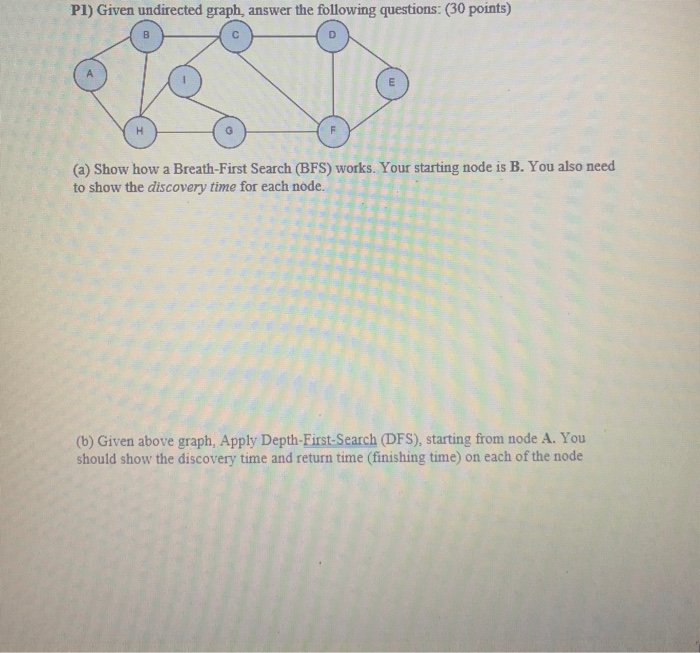PI) Given undirected graph answer the following questions: (30 points) (a) Show how a Breath-First Search (BFS) works. Your starting node is B. You also need to show the discovery time for each node. (b) Given above graph, Apply Depth-First-Search (DFS), starting from node A. You should show the discovery time and return time (finishing time) on each of the node

• ### 1. Startingatvertex000, perform a BFSof Q3.Assume all adjacency lists are in numericalorder.For example, (000,001) occurs before...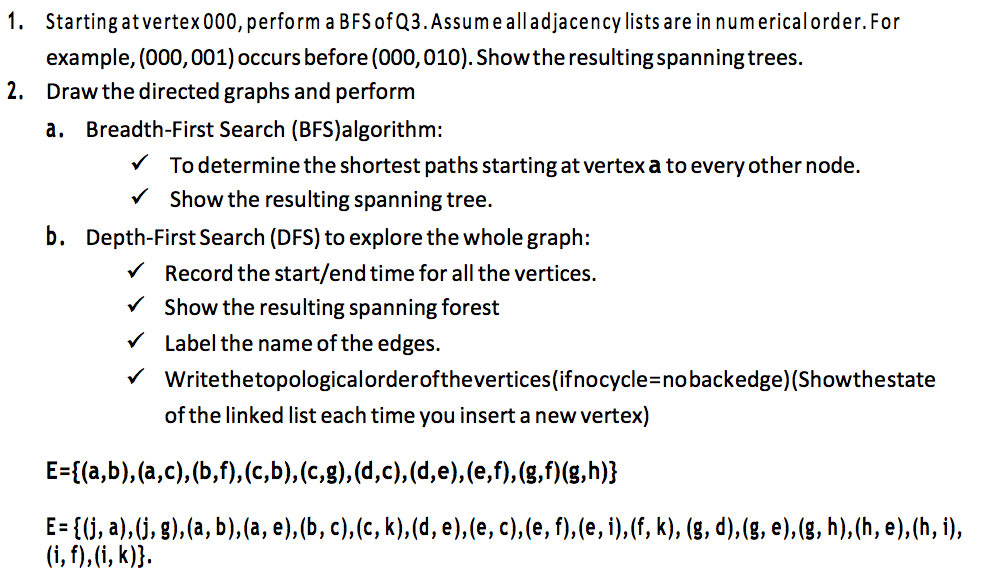1. Startingatvertex000, perform a BFSof Q3.Assume all adjacency lists are in numericalorder.For example, (000,001) occurs before (000, 010). Showthe resulting spanningtrees. Draw the directed graphs and perform a. 2. Breadth-First Search (BFS)algorithm: VTo determine the shortest paths starting at vertex a to everyother node. Show the resulting spanning tree. b. Depth-First Search (DFS) to explore the whole graph: Record the start/end time for all the vertices. show the resulting spanning forest Label the name°fthe edges. V Writethetopologicalorderofthevertices(ifnocycle-nobackedge) (Showthestate of the...

• ### 2. Consider the (undirected) graph G having the following vertex set Vand edge set E. V-0,1,2,3,4,5,6,7,8,9...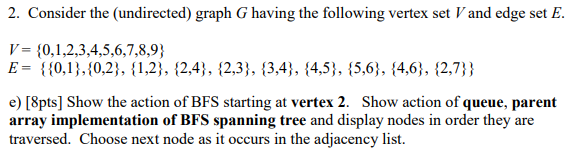2. Consider the (undirected) graph G having the following vertex set Vand edge set E. V-0,1,2,3,4,5,6,7,8,9 E- 0,1,10,2), 11,2;, 12,4), 12,3), 13,4), (4,5), {5.6,, 14,6, 2,7) e) [8pts] Show the action of BFS starting at vertex 2. Show action of queue, parent array implementation of BFS spanning tree and display nodes in order they are traversed. Choose next node as it occurs in the adjacency list.

• ### /* Graph read from file, and represnted as adjacency list. To implement DFS and BFS on...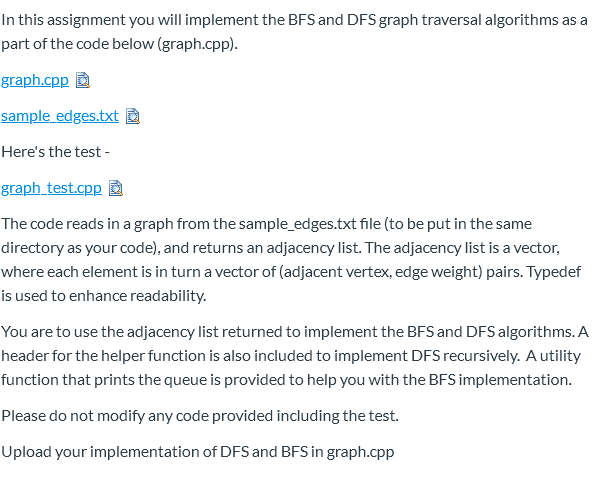/* Graph read from file, and represnted as adjacency list. To implement DFS and BFS on the graph */ #include <iostream> #include <sstream> #include <fstream> #include <vector> #include <utility> #include <unordered_map> #include <set> #include <queue> using namespace std; // Each vertex has an integer id. typedef vector<vector<pair<int,int>>> adjlist; // Pair: (head vertex, edge weight) adjlist makeGraph(ifstream& ifs); void printGraph(const adjlist& alist); vector<int> BFS(const adjlist& alist, int source); // Return vertices in BFS order vector<int> DFS(const adjlist& alist, int source); //...